#### 2.2Image Guide

This section introduces the 2htdp/image library through a series of increasingly complex image constructions and discusses some subtle details of cropping and outline images.

##### 2.2.1Overlaying, Above, and Beside: A House

To build a simple-looking house, we can place a triangle above a rectangle.

 > (above (triangle 40 "solid" "red") (rectangle 40 30 "solid" "black"))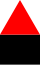We can give the house two roofs by putting two triangles next to each other.

 > (above (beside (triangle 40 "solid" "red") (triangle 40 "solid" "red")) (rectangle 80 40 "solid" "black"))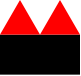But if we want the new roof to be a little smaller, then they do not line up properly.

 > (above (beside (triangle 40 "solid" "red") (triangle 30 "solid" "red")) (rectangle 70 40 "solid" "black"))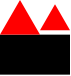Instead, we can use beside/align to line up the two triangles along their bottoms instead of along the middles (which is what beside does).

 > (define victorian (above (beside/align "bottom" (triangle 40 "solid" "red") (triangle 30 "solid" "red")) (rectangle 70 40 "solid" "black")))
> victorian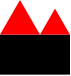To add a door to the house, we can overlay a brown rectangle, aligning it with the center bottom of the rest of the house.

> (define door (rectangle 15 25 "solid" "brown"))
> (overlay/align "center" "bottom" door victorian)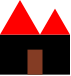We can use a similar technique to put a doorknob on the door, but instead of overlaying the doorknob on the entire house, we can overlay it just on the door.

 > (define door-with-knob (overlay/align "right" "center" (circle 3 "solid" "yellow") door))
> (overlay/align "center" "bottom" door-with-knob victorian)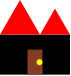##### 2.2.2Rotating and Overlaying: A Rotary Phone Dial

A rotary phone dial can be built by from a black disk and 10 little white ones by placing the white disks, one at a time, at the top of the black disk and then rotating the entire black disk. To get started, lets define a function to make little white disks with numbers on them:

 > (define (a-number digit) (overlay (text (number->string digit) 12 "black") (circle 10 "solid" "white")))

We’ll use place-and-turn to put the numbers onto the disk:

 > (define (place-and-turn digit dial) (rotate 30 (overlay/align "center" "top" (a-number digit) dial)))

For example:

 > (place-and-turn 0 (circle 60 "solid" "black"))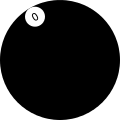> (place-and-turn 8 (place-and-turn 9 (place-and-turn 0 (circle 60 "solid" "black"))))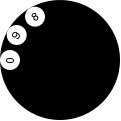We can write a single function to put all of the numbers together into the dial:

 > (define (place-all-numbers dial) (place-and-turn 1 (place-and-turn 2 (place-and-turn 3 (place-and-turn 4 (place-and-turn 5 (place-and-turn 6 (place-and-turn 7 (place-and-turn 8 (place-and-turn 9 (place-and-turn 0 dial)))))))))))
> (place-all-numbers (circle 60 "solid" "black"))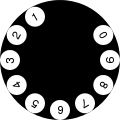That definition is long and tedious to write. We can shorten it using foldl:

 > (define (place-all-numbers dial) (foldl place-and-turn dial '(0 9 8 7 6 5 4 3 2 1)))
> (place-all-numbers (circle 60 "solid" "black"))To finish off the dial, we need to rotate it a little bit to its natural position and put a white disk in the center of it. Here’s the inner dial:

 > (define inner-dial (overlay (text "555-1234" 9 "black") (circle 30 "solid" "white")))

and here’s a function to build the entire rotary dial, with an argument that scales the dial:

 > (define (rotary-dial f) (scale f (overlay inner-dial (rotate -90 (place-all-numbers (circle 60 "solid" "black"))))))
> (rotary-dial 2)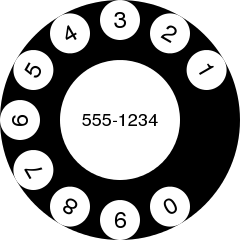Looking at the image, it feels like the numbers are too close to the edge of the dial. So we can adjust the place-and-turn function to put a little black rectangle on top of each number. The rectangle is invisible because it ends up on top of the black dial, but it does serve to push the digits down a little.

 > (define (place-and-turn digit dial) (rotate 30 (overlay/align "center" "top" (above (rectangle 1 5 "solid" "black") (a-number digit)) dial)))
> (rotary-dial 2)##### 2.2.3Alpha Blending

With shapes that have opaque colors like "red" and "blue", overlaying one on top completely blots out the one one the bottom.

For example, the red rectangle here completely covers the blue one.

 > (overlay (rectangle 60 100 "solid" (color 127 255 127)) (rectangle 100 60 "solid" (color 127 127 255)))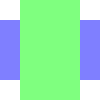But 2htdp/image also supports colors that are not completely opaque, via the (optional) fourth argument to color.

 > (overlay (rectangle 60 100 "solid" (color 0 255 0 127)) (rectangle 100 60 "solid" (color 0 0 255 127)))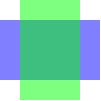In this example, the color (color 0 255 0 127) looks just like the color (color 127 255 127) when the background is white. Since white is (color 255 255 255), we end up getting 1/2 of 255 for the red and blue components and 255 for the green one.

We can also use alpha blending to make some interesting effects. For example, the function spin-alot takes an image argument and repeatedly places it on top of itself, rotating it each time by 1 degree.

 > (define (spin-alot t) (local [(define (spin-more i θ) (cond [(= θ 360) i] [else (spin-more (overlay i (rotate θ t)) (+ θ 1))]))] (spin-more t 0)))

Here are some uses of spin-alot, first showing the original shape and then the spun shape.

 > (rectangle 12 120 "solid" (color 0 0 255))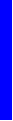> (spin-alot (rectangle 12 120 "solid" (color 0 0 255 1)))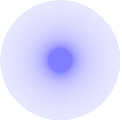> (triangle 120 "solid" (color 0 0 255))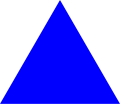> (spin-alot (triangle 120 "solid" (color 0 0 255 1)))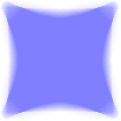> (isosceles-triangle 120 30 "solid" (color 0 0 255))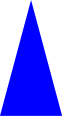> (spin-alot (isosceles-triangle 120 30 "solid" (color 0 0 255 1)))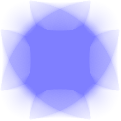##### 2.2.4Recursive Image Functions

It is also possible to make interesting looking shapes with little recursive functions. For example, this function repeatedly puts white circles that grow, evenly spaced around the edge of the given shape:

 > (define (swoosh image s) (cond [(zero? s) image] [else (swoosh (overlay/align "center" "top" (circle (* s 1/2) "solid" "white") (rotate 4 image)) (- s 1))]))
 > (swoosh (circle 100 "solid" "black") 94)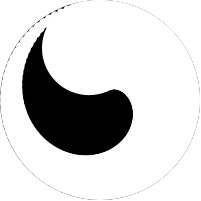More conventional fractal shapes can also be written using the image library, e.g.:

 > (define (sierpinski-carpet n) (cond [(zero? n) (square 1 "solid" "black")] [else (local [(define c (sierpinski-carpet (- n 1))) (define i (square (image-width c) "solid" "white"))] (above (beside c c c) (beside c i c) (beside c c c)))]))
 > (sierpinski-carpet 5)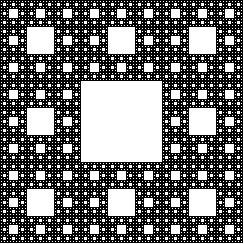> (define (colored-carpet colors) (cond [(empty? (rest colors)) (square 1 "solid" (first colors))] [else (local [(define c (colored-carpet (rest colors))) (define i (square (image-width c) "solid" (car colors)))] (above (beside c c c) (beside c i c) (beside c c c)))]))
 > (colored-carpet (list (color 51 0 255) (color 102 0 255) (color 153 0 255) (color 204 0 255) (color 255 0 255) (color 255 204 0)))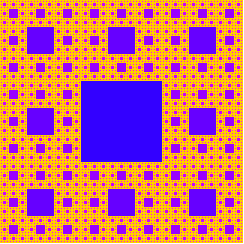The Koch curve can be constructed by simply placing four curves next to each other, rotated appropriately:

 > (define (koch-curve n) (cond [(zero? n) (square 1 "solid" "black")] [else (local [(define smaller (koch-curve (- n 1)))] (beside/align "bottom" smaller (rotate 60 smaller) (rotate -60 smaller) smaller))]))
> (koch-curve 5)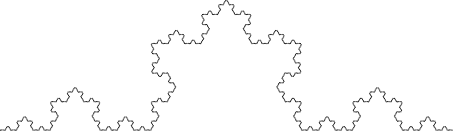And then put three of them together to form the Koch snowflake.

 > (above (beside (rotate 60 (koch-curve 5)) (rotate -60 (koch-curve 5))) (flip-vertical (koch-curve 5)))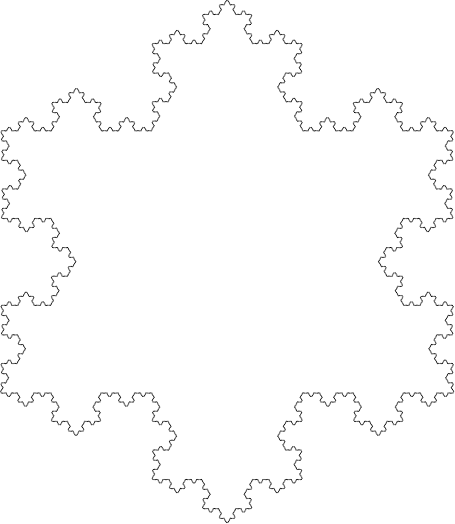##### 2.2.5The Nitty Gritty of Pixels, Pens, and Lines

The image library treats coordinates as if they are in the upper-left corner of each pixel, and infinitesimally small (unlike pixels which have some area).

Thus, when drawing a solid square of whose side-length is 10, the image library colors in all of the pixels enclosed by the square starting at the upper left corner of (0,0) and going down to the upper left corner of (10,10), so the pixel whose upper left at (9,9) is colored in, but the pixel at (10,10) is not. All told, 100 pixels get colored in, just as expected for a square with a side length of 10.

When drawing lines, however, things get a bit more complex. Specifically, imagine drawing the outline of that rectangle. Since the border is between the pixels, there really isn’t a natural pixel to draw to indicate the border. Accordingly, when drawing an outline square (without a pen specification, but just a color as the last argument), the image library uses a pen whose width is 1 pixel, but draws a line centered at the point (0.5,0.5) that goes down and around to the point (10.5,10.5). This means that the outline slightly exceeds the bounding box of the shape. Specifically, the upper and left-hand lines around the square are within the bounding box, but the lower and right-hand lines are just outside.

This kind of rectangle is useful when putting rectangles next to each other and avoiding extra thick lines on the interior. For example, consider building a grid like this:

> (define s1 (square 20 'outline 'black))
> (define r1 (beside s1 s1 s1 s1 s1 s1))
> (above  r1 r1 r1 r1 r1 r1)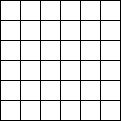The reason interior lines in this grid are the same thickness as the lines around the edge is because the rectangles overlap with each other. That is, the upper-left rectangle’s right edge is right on top of the next rectangle’s left edge.

The special case of adding 0.5 to each coordinate when drawing the square applies to all outline polygon-based shapes that just pass color, but does not apply when a pen is passed as the last argument to create the shape. For example, if using a pen of thickness 2 to draw a rectangle, we get a shape that has a border drawing the row of pixels just inside and just outside the shape. One might imagine that a pen of thickness 1 would draw an outline around the shape with a 1 pixel thick line, but this would require 1/2 of each pixel to be illuminated, something that is not possible. Instead, the same pixels are lit up as with the 2 pixel wide pen, but with only 1/2 of the intensity of the color. So a 1 pixel wide black pen object draws a 2 pixel wide outline, but in gray.

> (define p1 (make-pen "black" 1 "solid" "round" "round"))

> (rectangle 20 20 "outline" p1)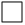When combining pens and cropping, we can make a rectangle that has a line that is one pixel wide, but where the line is drawn entirely within the rectangle. This rectangle has a two-pixel wide black pen, but we can crop out the outer portion of the pen.

> (define p2 (make-pen "black" 2 "solid" "round" "round"))
> (define s2 (crop 0 0 20 20 (rectangle 20 20 "outline" p2)))
> s2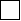Using that we can build a grid now too, but this grid has doubled lines on the interior.

> (define r2 (beside s2 s2 s2 s2 s2 s2))
> (above  r2 r2 r2 r2 r2 r2)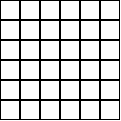While this kind of rectangle is not useful for building grids, it is important to be able to build rectangles whose drawing does not exceed its bounding box. Specifically, this kind of drawing is used by frame and empty-scene so that the extra drawn pixels are not lost if the image is later clipped to its bounding box.

When using image->color-list with outline shapes, the results can be surprising for the same reasons. For example, a 2x2 black, outline rectangle consists of nine black pixels, as discussed above, but since image->color-list only returns the pixels that are within the bounding box, we see only three black pixels and one white one.

 > (image->color-list (rectangle 2 2 "outline" "black"))
 (list (color 0 0 0 255) (color 0 0 0 255) (color 0 0 0 255) (color 255 255 255 0))

The black pixels are (most of) the upper and left edge of the outline shape, and the one white pixel is the pixel in the middle of the shape.